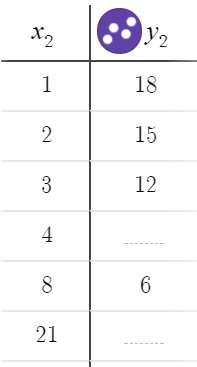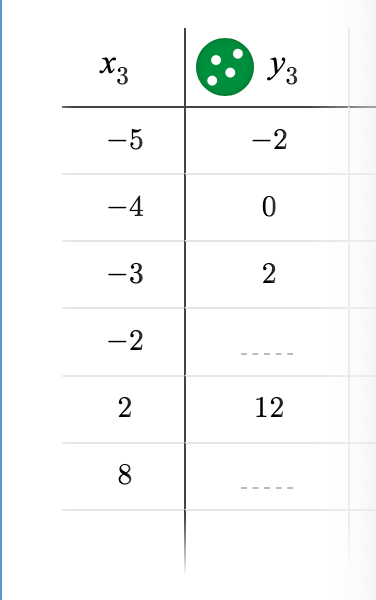# LINR 1 | Lesson 1 | Practice

Complete each section to show your understanding for linear patterns.

## Tables

The following tables represent linear patterns. The tables are created using Desmos.com.1. Find the missing values in each table.
2. Graph each table on an $$x$$-$$y$$ coordinate plane.
3. Calculate the slope using the table.
4. Calculate the slope using the graph.
5. Determine the point of the $$y$$intercept
6. Write an equation to determine the $$y$$value given any $$x$$-value.

## Patterns

Given the growth pattern shown in Figure 2 and Figure 4.1. Draw Figure 3.
2. Explain the growth pattern.
3. Determine the number of squares in Figure 0.
4. Write the equation to represent the number of squares for any figure.
5. Using the context of growth patterns, explain why $$m$$ represents the slope and $$b$$ represents the $$y$$-intercept in the slope-intercept formula $$y=mx+b$$.

A given linear pattern has 10 squares in Step 3.  The pattern grows by -4 squares every 2 steps.

1. Create a table for this pattern.
2. Calculate the slope to represent the growth for this pattern.
3. Calculate the equation to calculate the number of squares for each step.